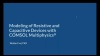## Transient and Frequency-Domain Analyses of Devices with Resistance and Capacitance

In Part 6 of this 10-part course on modeling resistive and capacitive devices, we turn our focus to the modeling of time-varying fields of resistive and capacitive devices. We start by briefly discussing how the behavior of such a system is governed by a time constant and then begin building a simple model of a device with resistance and capacitance, from which we compute the time constant for the DC case. After evaluating the model under DC conditions, we then take this model into the time domain. We add a new, second, time-dependent study; solve for the conduction currents and displacement currents for the time-varying simulation; and show how to check our transient solution. Thereafter, we show how to compare the results to the initial, DC simulation in the first study using the withsol operator.

We then show two ways to introduce a variation of the fields over time:

1. By incorporating a Boolean expression in the Terminal boundary condition node's settings, which is a more simple implementation, but one that involves taking many time steps
2. By using the Events interface, which uses significantly less time steps

We also show how to introduce a periodic variation of the fields over time and then walk through how to extract certain quantities from the results, namely the electric losses of the system over time. After, we show how to obtain the cycle-averaged heating losses out of the system three different ways:

1. Through using the time average operator
2. By monitoring the deposited energy in the system over time through adding the Global ODEs and DAEs interface
3. Through taking the solution and converting it from the time domain to the frequency domain

Lastly, we end with a detailed discussion of the governing equations being solved for in these types of models and how the time domain or frequency domain can be used to reconstruct the solution over time.